﻿ Numerical Modelling Study of the Effect of Artificial Cementation on the Mechanical Behaviour of Sandy SoilPublications are Open
Access in this journal
Article Versions
Export Article
• Normal Style
• MLA Style
• APA Style
• Chicago Style
Open Access Peer-reviewed

### Numerical Modelling Study of the Effect of Artificial Cementation on the Mechanical Behaviour of Sandy Soil

Abdulla AL-Rashidi , Saad Almutairi, Talal Almutairi
American Journal of Civil Engineering and Architecture. 2022, 10(1), 8-15. DOI: 10.12691/ajcea-10-1-2
Received January 15, 2022; Revised February 18, 2022; Accepted February 27, 2022

### Abstract

Soil stabilization and improvement are important issues in the field of geotechnical engineering. The use of artificial cementation has proven to be one of the most effective methods for resolving geotechnical engineering problems, including soil improvement techniques. In this study, the behaviour of artificially cemented sand is examined with the finite element method, using COMSOL Multiphysics 5.6 software. The influence on mechanical behaviour when adding different amounts of Portland cement is numerically investigated. The results showed induced cementation between soil particles can be realized by increasing strength parameters such as cohesion and angle of internal friction, which denote the stress-strain behaviour of tested soil samples. In addition, the use of Portland cements has a significant impact on the soil’s behaviour in terms of plastic strain. It is also recommended to apply the soil stabilization methods to deal with various geotechnical aspects such as weak soil layers and slope stability issues.

### 1. Introduction

In general, fieldwork associated with construction work is subject to and highlights multiple challenges. One example of such a challenge is when the soil sampled at a proposed site is not suitable for supporting the loads that will be imposed by the proposed structure. The role of the geotechnical engineer is to address this by determining the best solutions in terms of cost and quality, while not raising additional safety concerns on the site. In this regard, there are multiple techniques and methods which can be implemented to deal with each case, employing appropriate techniques and methods. The most commonly cited techniques are compaction, preloading, reinforcement, stabilization, etc. In this study, stabilization using different amounts of Portland cement will be investigated in depth.

Soil improvement, and or soil stabilization, are central topics in the field of geotechnical engineering, as they are considered efficient solutions for resolving the geotechnical problems associated with the implementation of civil engineering projects, which are directly related to soil properties and soil behaviour. The principal objective when using artificial cementation is to increase the strength, compressibility and permeability coefficient of the soil particles.

In the Kuwaiti context, there are many practical applications for artificial cementation, using different compositions of Portland cement as an additive. For example, transportation infrastructure construction projects and slope embankment protection 1, 2. Moreover, there are a large number of published studies investigating the roles for both natural and artificial cementation when adding cement in small amounts ranging from 1% to 5% by weight to enhance the soil properties of sand particles 2, 3, 4, 5, 6. Cementation in sand particles can be achieved by adding cementing agents such as silica and carbonates 4. However, both naturally and artificially cemented sand is vulnerable to brittle mode failure under low confining pressure and ductile mode failure under high confining pressure. The cementation of soil particles can have a significant effect on the strength parameters (cohesion and the internal friction angle) of the soil, which will lead to a systematic increase in soil stiffness and brittleness criterions 7. In their research, 8 studied the behaviour of artificially cemented carbonate sand and found that artificial cementation reduces specific volume, as a result of the greater number of fine particles in the soil sample.

Various types of cemented sands are available in Kuwait and Gulf cooperation countries, all naturally created from the precipitation of salts into the ground as a result of rainfall 2, 9, 10, 11. In particular, in Kuwait, there is a high demand for cement stabilization to address frequent soil problems associated with construction works, such as those related to replacing or stabilizing soft or loose soil layers to achieve larger weight bearing capacity in cases of relatively small deformations 2. In this study, the effect of using 1%, 2%, or 4% by weight of Portland cement on sand in Kuwait will be investigated using experimental tests, including a triaxial test. In addition, the behaviour of soil will be investigated numerically applying finite element methods. The focus will be directed towards investigating the behaviour of soil to achieve adequate yield and plastic strain values. The plastic deformation of soil grains describes important issues associated with postulated micro-mechanisms that have been derived and applied 12.

This study will be conducted using the Mohr-coulomb constitutive model, which will provide an adequate means to investigate the effects of Portland cement additives once added, and will also provide an important view point regarding numerical modelling analysis taking into consideration other variable parameters, such as using different confining pressures to apply deviator stress to soil samples.

### 2. Methodology

2.1. Testing Program

The data collected for this study, including that detailing experimental results pertaining to soil properties, was taken from 2, which describes sandy soil samples extracted from a depth of 1.0 to 1.5 m in the Umalhiman area of the southern part of Kuwait. The artificially cemented soil samples were prepared in a laboratory, with the desired amount of cement added to proper amount of sand for each specimen. 1%, 2%, 4% weights of Portland cement were added to the samples in order to create artificial cemented sands. The fine particles constitute 10.2% of the total soil particles, and the mean diameter of the soil particles is 0.32. The soil distribution curve is shown in Figure 1, and a summary of these soil properties is listed in Table 1.

The main objective of this research is to numerically investigate the effect of artificial cementation on the mechanical behaviour of the soil samples using a finite element method based on COMSOL Multiphysics 5.6 software. The software allows for a wide range of engineering problems to be simulated and analysed. For this study, the structural mechanics physics and stationery physics interfaces will be employed.

The use of numerical modelling techniques is an important, effective and helpful step. It provides a wide range of options that researchers can benefit from when developing their research objectives, instead of having to rely solely on the available experimental results. One of the chief components of numerical modelling is the validation stage. This stage will assist in finding the appropriate model and identify an effective model for use in the proposed modelling geometry 13.

The triaxial experimental results collated by 2 will be validated using the proposed numerical model for this study, which shares the same geometry and soil properties. The main experimental results for the drained triaxial tests are listed in Table 2, and the proposed geometry is shown in Figure 2. After conducting the simulation, the results will be obtained and then compared with those obtained from the experimental results. After this, the model will be extended to fulfil the objectives of this study by conducting a more advanced analysis.

• Figure 1. Grain size distribution curve (data collected from )
• Figure 2. Geometric configuration of the proposed model
2.2. Numerical Model

In addition to collecting data from experimental tests, numerical modelling is chiefly employed in research to achieve the study objectives. The numerical modelling will be conducted using COMSOL Multiphysics software. This software is widely used to resolve finite elements problems, and has a wide range of features and different physics modules that enable users to use it smoothly 14.

As stated above, the study conducted by 2 will be considered for validation purposes in this study. 2 used cylindrical samples with a 71 mm diameter and a 150 mm in length. He conducted a series of consolidation undrained triaxial tests at 100 kPa, 200 kPa, 300 kPa and 400 kPa.

The proposed model geometry is shown in Figure 2. A 2-D geometry is modelled implementing similar validation study dimensions. In addition, the same properties as those reported in the validation study were employed, and a Mohr-coulomb constitutive model applied for the used sand. Deviator stresses and confining stresses, from two different numerical investigation cases at 100 kPa and 200 kPa, were applied to simulate the triaxial test on the soil sample. After validating the numerical model by comparing the results from both the experimental and the numerical modelling and having attained acceptable results, the numerical model was extended to ascertain the study objectives. This involved applying different parameters and loads to investigate the effects of artificial cementation using different Portland cement amounts as stated above, to establish the mechanical behaviour of the sand. To establish the boundaries of the proposed numerical model (see Figure 2), a fixed base geometry was used to prevent movement in both the vertical and horizontal directions, and a prescribed displacement applied to the upper part of the geometry to enable the software to calculate the stresses with different displacements up to the greatest displacement. Finally, the model was meshed with mapped meshing (Figure 3).

• Figure 3. Finite elements mesh

Symmetry is considered for geometry as it saves computational time from the simulation using COMSOL Multiphysics 5.6 software. A 2-D mapped meshing format was assumed for meshing.

2.3. Soil Behaviour

The best and most effective way to study the mechanical behaviours of engineering materials and specifically soil is illustrated with the stress-strain diagram. The effect of applied loads on soil samples (the confining pressure and deviator stress) will be shown in the stress-strain diagram from the point of first applying loads, passing through ultimate stress and ending with the failure point, at which the sample will collapse. Moreover, the properties and the characteristic of the constitutive model employed will be presented. For example, the Mohr-coulomb constitutive model which is widely used to model the behaviour of soil in different engineering practices will be the principal guide for analysing the results obtained in the stress-strain diagram. The main Mohr-coulomb constitutive model parameters are cohesion and internal friction angles. Both parameters will have primary effects on the mechanical behaviour of the soil samples, certainly when using differing amounts of Portland cement materials. This will be discussed in detail in the results and discussion sections of this research.

According to plasticity theory, effective strain or shear strain intensity represents the total deformation and or distortion of material elements 15, 16. The unrecoverable area after the yield limit of the strain is reached is called effective plastic strain 17. In terms of the elastic strain behaviour of soil; when the applied stress reaches the failure point the stress is constant, whereas in cases of plastic strain behaviour, the strain would behave differently. In other words, the strain or certainly the plastic strain would remain constant, decreasing or increasing according to the soil’s properties. This would provide an advanced opportunity to focus on the post-failure behaviour of the soil. In geotechnical engineering, predicting the post-failure behaviour of soil is important, especially, when considering the boundary value practices for different geotechnical modelling applications, such as the failure at different points under a footing 18.

Total strain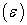is determined by the following equation 18: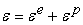(1)

Where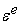and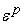are elastic and plastic strain, respectively.

### 3. Results and Discussions

The numerical validation component of this study is shown in Figure 4. The validation was conducted at two different confining pressures 100 kPa and 200 kPa in the experimental undrained triaxial tests. It can be seen that the graphs for both the experimental and numerical modelling show high consistency and agreement, with small variances ranging between 0.95% and 4.92%. This falls within an acceptable range for many finite element modelling applications.

After validating the experimental results, the numerical model was extended and simulated with COMSOL Multiphysics software. The stress-strain curves were determined by simulating drained triaxial tests that represent axial strain versus deviator stress with different confining pressures, as well as considering different Portland cement amounts, as presented in Figure 5.

• Figure 4. Validation of the proposed model
• Figure 5. Deviator stress versus Axial strain for different cement amounts and confining pressures
• Figure 6. Stress path for different confining pressures

Deviator stress is calculated by the following: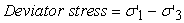(2)

Where σ'1 is the vertical force and σ'3 is the horizontal force (confining pressure)

From Figure 5, it can be noted that all cases behave in a similar pattern, starting with an elastic linear line, and then at a certain point starting with plastic behaviour with constant plastic strain. This can be explained by employing a constitutive model (Mohr-coulomb) that is widely used for representing the behaviour of soil in different engineering practices. In addition, the effect of confining pressure magnitudes, as well as the Portland cement additives is clear. It can be described thus; as the confining pressure increases the stress deviators induced in the soil sample increase, thereby increasing the cement additives.

The stress path was determined from the numerical modelling results of the tested soil according to the following equations 19: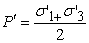(3)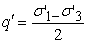(4)

Figure 6 represents the stress path of the numerical model for the tested sand with different cement contents and different confining pressures. The failure envelopes are linear, and where the effect of adding cement to the soil is clear, the soil failure envelope increased with greater amounts of cement.

The effect of confining pressure and, specifically, use of artificial cementation, on soil behaviour in terms of plastic strain is clearly represented in Figure 7 and Figure 8.

From these Figures it is apparent that increasing the confining pressure led to a reduction in the plastic regions of the soil samples and the use of artificial cementation in differing amounts has a significant effect on minimising the plastic regions in the soil samples tested. Moreover, the use of 4% Portland cement totally eliminated the effect of plastic strain in the soil samples, which would otherwise be explained by the high strength of the soil resulting from the high cementation bond. This would also require greater focus to be placed on the effect of the amount of used cement on soil sample cohesion.

It is generally accepted that it is reasonable to examine the type of strain-stress by evaluating the behaviour of the tested material for ductility and brittleness criterions under the applied load. This is essential when dealing with steel or concrete materials. For soil and specifically sand, and in this study where artificial cementation is directly affecting strength parameters, particularly cohesion, it is important to look at the brittleness property in relation to different cement formulations. This will give a clear indication of the effect of using different cement formulations on the ductility properties of the tested sand samples. For this purpose, the brittleness index (IB) is calculated using the following equation 20, 21: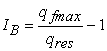(5)

Where, qf and qu are the peak and residual stresses, respectively.

The calculated brittleness index is 0.435, 0.687 and 2.976 when using 1%, 2% and 4% of Portland cement with soil samples respectively. Hence, artificial cementation has a significant effect on the tested soil samples in terms of increasing their ductility.

• Figure 7. Plastic strain for different cement contents for deviator stress of 20 kPa and 40 kPa
• Figure 8. Plastic strain for different cement contents for deviator stress of 80 kPa and 160 kPa

### 4. Conclusions

The effects when using artificial cementation on the mechanical behaviour of Kuwaiti soil were investigated using the numerical modelling software, COMSOL Multiphysics 5.6. The data and key soil properties and parameters were taken from a previously published paper 2. The experimental data was simulated and validated using the proposed model, and then the model was extended by changing selected variables and parameters to achieve the aim of this study. The stress-strain curves represented increased soil stiffness as a consequence of using particular cement contents, and the effect of increasing the confining pressures was also important. When testing soil samples of different cement contents and different confining pressures, the same behaviour was present both before the yield point in the elastic region and beyond the yield point in the plastic region; the only difference related to soil stiffness and peak stress envelops. The effect of artificial cementation was significant for decreasing the area of plastic regions induced, and eliminating this effect when using a 4% cement content with the highest confining pressure. In addition, the ductility of the tested soil samples generated a positive response with regard to the use of artificial cementation by means of increasing ductility. In conclusion, the effect of artificial cementation using 1%, 2% and 4% Portland cement is significant for improving the properties of Kuwaiti soils. It is also advised to use this method for soil stabilization when addressing various geotechnical problems such as weak soil layers and challenges with slope stability.

### References

  N. F. Ismael, “Cement stabilization of kuwaiti soils,” Arab gulf J. Sci. Res., vol. 2, no. 1, 1984. In article  N. F. Ismael, “Inflence of artificial cementation on the properties of Kuwaiti sands,” Kuwait J. Sci. Eng., vol. 27, no. 1, 2000. In article  S. H. E Asghari, DG Toll, “Effect of cementation on the shear strength of Tehran gravelly sand using triaxial tests,” J. Sci. Islam. Repub. Iran, vol. 15, no. 1, pp. 65-72, 2004. In article  G. W. Clough, N. Sitar, and R. C. Bachus, “Cemented Sands Under Static Loading,” J. Geotech. Eng. Div., vol. 107, no. 6, pp. 799-817, 1981. In article View Article  A. Diambra, L. Festugato, E. Ibraim, A. Peccin da Silva, and N. C. Consoli, “Modelling tensile/compressive strength ratio of artificially cemented clean sand,” Soils Found., vol. 58, no. 1, pp. 199-211, 2018. In article View Article  Y. H. Wang and S. C. Leung, “Characterization of cemented sand by experimental and numerical investigations,” J. Geotech. Geoenvironmental Eng., vol. 134, no. 7, pp. 992–1004, 2008. In article View Article  S. M. Haeri, A. Hamidi, S. M. Hosseini, E. Asghari, and D. G. Toll, “Effect of cement type on the mechanical behavior of a gravely sand,” Geotech. Geol. Eng., vol. 24, no. 2, pp. 335-360, 2006. In article View Article  M. R. Coop and J. H. Atkinson, “The mechanics of cemented carbonate sands,” Geotechnique, vol. 43, no. 1, pp. 53-67, 1993. In article View Article  N. Ismael, M. Mollah, and O. Al-khalidi, “Geotechnical properties of cemented soils in Kuwait,” Aust. Road Res., vol. 16, no. 2, pp. 94-104, 1986. In article  N. F. Ismael, “Loading tests on circular and ring plates in very dense cemented sands,” J. Geotech. Geoenvironmental Eng., vol. 122, pp. 281-287, 1996. In article View Article  N. F. Ismael, “PROPERTIES AND BEHAVIOR OF CEMENTED SAND DEPOSITS IN KUWAIT,” Soils Found., vol. 39, no. 4, pp. 47-57, 1999. In article View Article  Y. P. Cheng, M. D. Bolton, and Y. Nakata, “Crushing and plastic deformation of soils simulated using DEM,” Geotechnique, vol. 54, no. 2, pp. 131-141, 2004. In article View Article  R. Fellows and A. Liu, Research Methods for Construction, vol. 3, no. 2. A John Wiley & Sons. Ltd, Publication, 2015. In article  J. Hickey and J. Gottsmann, “Benchmarking and developing numerical Finite Element models of volcanic deformation,” J. Volcanol. Geotherm. Res., vol. 280, pp. 126–130, 2014. In article View Article  L. KACHANOV, Fundamentals of the Theory of Elasticity. New York: Dover Publications, 2004. In article  X. Ding and G. Zhang, “Coefficient of equivalent plastic strain based on the associated flow of the Drucker-Prager criterion,” Int. J. Non. Linear. Mech., vol. 93, pp. 15-20, 2017. In article View Article  M. C. M. Nasvi, P. G. Ranjith, and J. Sanjayan, “A numerical study of triaxial mechanical behaviour of geopolymer at different curing temperatures: An application for geological sequestration wells,” J. Nat. Gas Sci. Eng., vol. 26, pp. 1148-1160, 2015. In article View Article  A. M. Puzrin, Constitutive Modelling in Geomechanics, vol. 53, no. 9. Springer, 2012. In article View Article  B. M. Das, Principles of Foundation Engineering, Fourth edi. Cole Publishing Company, 1999. In article  N. C. Consoli, P. D. M. Prietto, and L. A. Ulbrich, “Influence of Fiber and Cement Addition,” J. Geotech. Geoenvironmental Eng., vol. 124, no. 12, pp. 1211-1214, 1998. In article View Article  S. S. Kutanaei and A. J. Choobbasti, “Triaxial behavior of fiber-reinforced cemented sand,” J. Adhes. Sci. Technol., vol. 30, no. 6, pp. 579-593, 2016. In article View ArticleThis work is licensed under a Creative Commons Attribution 4.0 International License. To view a copy of this license, visit http://creativecommons.org/licenses/by/4.0/